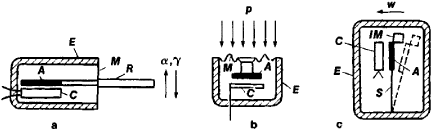# Mechanotron

The following article is from The Great Soviet Encyclopedia (1979). It might be outdated or ideologically biased.

## Mechanotron

an electronic vacuum device in which the strength of an electron or ion current is controlled by the direct mechanical displacement of the electrodes of the device. Mechanotrons are designed to convert mechanical quantities into electrical quantities and are used extensively as sensors (transducers) for the measurement of such quantities as displacements from 0.01 to 100 microns (μ); forces from 1 micronewton (μN) to 1 newton (N) (Figure 1, a), pressures from 0.1 N/m2 to 1 MN/m2 (Figure 1, b), accelerations from 0.001 to 100 m/ sec2, and vibrations having frequencies up to 10 kilohertz (Figure 1, c).Figure 1. Principal types of mechanotrons: (a) for displacement and force measurements, (b) for pressure measurements, (c) for acceleration and vibration measurements. (A) Movable anode, (C) fixed cathode, (E) envelope, (M) flexible membrane (or sylphon bellows) rigidly connected to the anode, (R) control rod soldered to the membrane, (S) flat spring, (IM) inertial mass attached to the movable electrode. Arrows indicate the direction of action of the mechanical signal: displacement (a), force (7), pressure (p), acceleration (w).

A characteristic feature of the mechanotron is that the displacement of one or more movable electrodes (for example, an anode) with respect to the fixed cathode causes a change in the value and configuration of the electric field between the electrodes and thus a change in the strength of the anode current. The total number of electrodes may be two (diode), three (triode), or four (tetrode). The most common type of mechanotron is the diode mechanotron, which usually has a dual design (a fixed cathode and two movable anodes) and is used in bridge measuring circuits. The chief advantages of mechanotron transducers are the high current sensitivity (up to 7 A/cm for diode mechanotrons), the high voltage sensitivity (up to 25 kV/cm), the high stability and reliability of the readings, the simplicity of the design and circuitry, the small size, and the low weight.

### REFERENCE

Berlin, G. S. Elektronnye pribory s mekhanicheski upravliaemymi elektrodami. Moscow, 1971.

G. S. BERLIN